• 累计撰写 13 篇文章
• 累计收到 9 条评论

# numpy基础2021-11-26 / 1 评论 / 12 阅读 / 正在检测是否收录...

NumPy是Python中科学计算的基础包。它是一个Python库，提供多维数组对象，各种派生对象（如掩码数组和矩阵），以及用于数组快速操作的各种API，有包括数学、逻辑、形状操作、排序、选择、输入输出、离散傅立叶变换、基本线性代数，基本统计运算和随机模拟等等。

NumPy包的核心是 ndarray 对象。它封装了python原生的同数据类型的 n 维数组，为了保证其性能优良，其中有许多操作都是代码在本地进行编译后执行的。

## 生成NumPy数组

NumPy是Python的外部库，不在标准库中。因此，若要使用它，需要先导入NumPy。

import numpy as np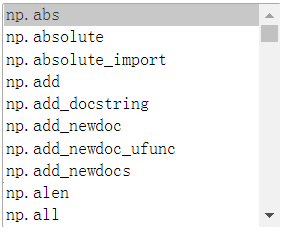### 利用random模块生成数组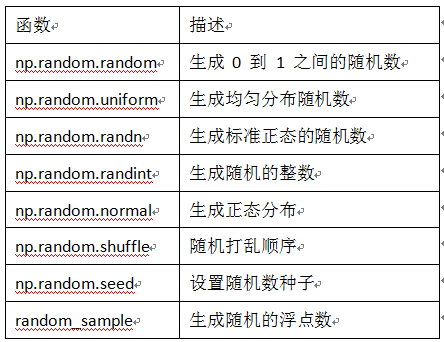### 创建特定形状的多维数组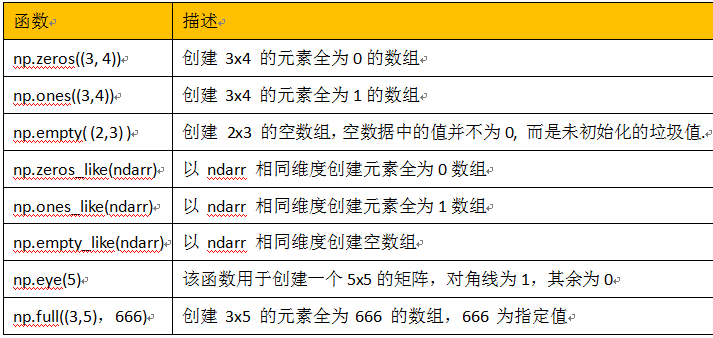### 利用 arange、linspace 函数生成数组

arange 是 numpy 模块中的函数，其格式为:

arange([start,] stop[,step,], dtype=None)

import numpy as np
print(np.arange(10))
# [0 1 2 3 4 5 6 7 8 9]
print(np.arange(0, 10))
# [0 1 2 3 4 5 6 7 8 9]
print(np.arange(1, 4, 0.5))
# [1.  1.5 2.  2.5 3.  3.5]
print(np.arange(9, -1, -1))
# [9 8 7 6 5 4 3 2 1 0]

linspace 也是 numpy 模块中常用的函数，其格式为:

np.linspace(start, stop, num=50, endpoint=True, retstep=False, dtype=None)

import numpy as np

print(np.linspace(0, 1, 10))
#[0.         0.11111111 0.22222222 0.33333333 0.44444444 0.55555556
# 0.66666667 0.77777778 0.88888889 1.        ]

## NumPy的算术运算

### 对应元素相乘

numpy.multiply(x1, x2, /, out=None, *, where=True,casting='same_kind', order='K', dtype=None, subok=True[, signature, extobj])

A = np.array([[1, 2], [-1, 4]])
B = np.array([[2, 0], [3, 4]])
A*B
##结果如下：
array([[ 2,  0],
[-3, 16]])
#或另一种表示方法
np.multiply(A,B)
#运算结果也是
array([[ 2,  0],
[-3, 16]])

### 点积运算

numpy.dot(a, b, out=None)

X1=np.array([[1,2],[3,4]])
X2=np.array([[5,6,7],[8,9,10]])
X3=np.dot(X1,X2)
print(X3)
print(X1@X2)

### 线性代数

import numpy as np
a = np.array([[1.0, 2.0], [3.0, 4.0]])
print(a)
print(a.transpose())
print(np.linalg.inv(a)) # 矩阵求逆
print(np.eye(2)) # unit 2x2 matrix; "eye" represents "I" 单位矩阵
print(np.trace(a))  # trace

y = np.array([[5.], [7.]])
np.linalg.solve(a, y) #矩阵的解 ax=y x的解
np.linalg.eig(j) # 矩阵特征向量

## 数组变形

### 更改数组的形状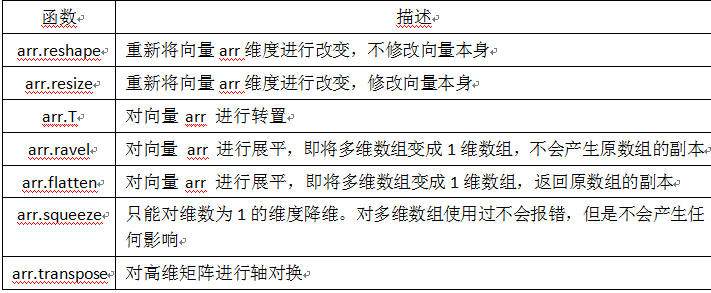>>> a = np.floor(10*np.random.random((3,4)))
>>> a
array([[ 2.,  8.,  0.,  6.],
[ 4.,  5.,  1.,  1.],
[ 8.,  9.,  3.,  6.]])
>>> a.shape
(3, 4)

### 合并数组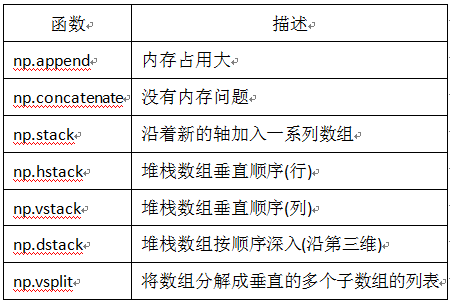（1）append 合并一维数组

import numpy as np

a =np.array([1, 2, 3])
b = np.array([4, 5, 6])
c = np.append(a, b)
print(c)
# [1 2 3 4 5 6]

import numpy as np

a =np.arange(4).reshape(2, 2)
b = np.arange(4).reshape(2, 2)
# 按行合并
c = np.append(a, b, axis=0)
print('按行合并后的结果')
print(c)
print('合并后数据维度', c.shape)
# 按列合并
d = np.append(a, b, axis=1)
print('按列合并后的结果')
print(d)
print('合并后数据维度', d.shape)

（2）concatenate 沿指定轴连接数组或矩阵

import numpy as np
a =np.array([[1, 2], [3, 4]])
b = np.array([[5, 6]])

c = np.concatenate((a, b), axis=0)
print(c)
d = np.concatenate((a, b.T), axis=1)
print(d)

（3）stack 沿指定轴堆叠数组或矩阵

import numpy as np

a =np.array([[1, 2], [3, 4]])
b = np.array([[5, 6], [7, 8]])
print(np.stack((a, b), axis=0))

### 数组的性质

• ndarray.ndim - 数组的轴（维度）的个数。在Python世界中，维度的数量被称为rank。
• ndarray.shape - 数组的维度。这是一个整数的元组，表示每个维度中数组的大小。对于有 n 行和 m 列的矩阵，shape 将是 (n,m)。因此，shape 元组的长度就是rank或维度的个数 ndim
• ndarray.size - 数组元素的总数。这等于 shape 的元素的乘积。
• ndarray.dtype - 一个描述数组中元素类型的对象。可以使用标准的Python类型创建或指定dtype。另外NumPy提供它自己的类型。例如numpy.int32、numpy.int16和numpy.float64。
• ndarray.itemsize - 数组中每个元素的字节大小。例如，元素为 float64 类型的数组的 itemsize 为8（=64/8），而 complex32 类型的数组的 itemsize 为4（=32/8）。它等于 ndarray.dtype.itemsize
• ndarray.data - 该缓冲区包含数组的实际元素。通常，我们不需要使用此属性，因为我们将使用索引访问数组中的元素。
>>> import numpy as np
>>> a = np.arange(15).reshape(3, 5)
>>> a
array([[ 0,  1,  2,  3,  4],
[ 5,  6,  7,  8,  9],
[10, 11, 12, 13, 14]])
>>> a.shape
(3, 5)
>>> a.ndim
2
>>> a.dtype.name
'int64'
>>> a.itemsize
8
>>> a.size
15
>>> type(a)
<type 'numpy.ndarray'>
>>> b = np.array([6, 7, 8])
>>> b
array([6, 7, 8])
>>> type(b)
<type 'numpy.ndarray'>

## 批量处理

import numpy as np
#生成10000个形状为2X3的矩阵
data_train = np.random.randn(10000,2,3)
#这是一个3维矩阵，第一个维度为样本数，后两个是数据形状
print(data_train.shape)
#(10000,2,3)
#打乱这10000条数据
np.random.shuffle(data_train)
#定义批量大小
batch_size=100
#进行批处理
for i in range(0,len(data_train),batch_size):
x_batch_sum=np.sum(data_train[i:i+batch_size])
print("第{}批次,该批次的数据之和:{}".format(i,x_batch_sum))

## 通用函数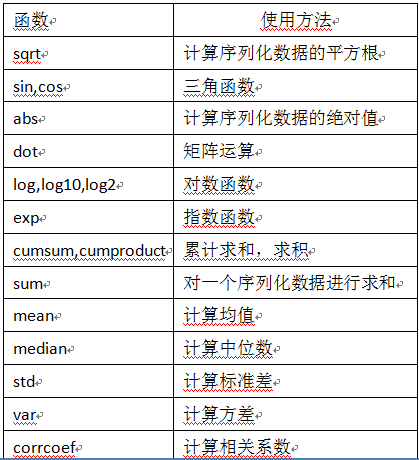math与numpy函数的性能比较：

import time
import math
import numpy as np

x = [i * 0.001 for i in np.arange(1000000)]
start = time.time()
for i, t in enumerate(x):
x[i] = math.sin(t)
print ("math.sin:", time.time() - start )

x = [i * 0.001 for i in np.arange(1000000)]
x = np.array(x)
start = time.time()
np.sin(x)
print ("numpy.sin:", time.time() - start )
math.sin: 0.45232319831848145
numpy.sin: 0.017247676849365234

## 拷贝和视图

### 完全不复制

>>> a = np.arange(12)
>>> b = a            # no new object is created
>>> b is a           # a and b are two names for the same ndarray object
True
>>> b.shape = 3,4    # changes the shape of a
>>> a.shape
(3, 4)

Python将可变对象作为引用传递，因此函数调用不会复制。

>>> def f(x):
...     print(id(x))
...
>>> id(a)                           # id is a unique identifier of an object
148293216
>>> f(a)
148293216

### 视图或浅拷贝

>>> c = a.view()
>>> c is a
False
>>> c.base is a                        # c is a view of the data owned by a
True
>>> c.flags.owndata
False
>>>
>>> c.shape = 2,6                      # a's shape doesn't change
>>> a.shape
(3, 4)
>>> c[0,4] = 1234                      # a's data changes
>>> a
array([[   0,    1,    2,    3],
[1234,    5,    6,    7],
[   8,    9,   10,   11]])

>>> s = a[ : , 1:3]     # spaces added for clarity; could also be written "s = a[:,1:3]"
>>> s[:] = 10           # s[:] is a view of s. Note the difference between s=10 and s[:]=10
>>> a
array([[   0,   10,   10,    3],
[1234,   10,   10,    7],
[   8,   10,   10,   11]])

### 深拷贝

copy方法生成数组及其数据的完整副本。

>>> d = a.copy()                          # a new array object with new data is created
>>> d is a
False
>>> d.base is a                           # d doesn't share anything with a
False
>>> d[0,0] = 9999
>>> a
array([[   0,   10,   10,    3],
[1234,   10,   10,    7],
[   8,   10,   10,   11]])

>>> a = np.arange(int(1e8))
>>> b = a[:100].copy()
>>> del a  # the memory of a can be released.

## 广播机制

NumPy的Universal functions 中要求输入的数组shape是一致的，当数组的shape不相等的时候，则会使用广播机制。不过，调整数组使得shape一样，需满足一定规则，否则将出错。这些规则可归结为以下四条：

• 让所有输入数组都向其中shape最长的数组看齐，shape中不足的部分都通过在前面加1补齐； 如：a：2x3x2 b：3x2，则b向a看齐，在b的前面加1：变为：1x3x2
• 输出数组的shape是输入数组shape的各个轴上的最大值；
• 如果输入数组的某个轴和输出数组的对应轴的长度相同或者其长度为1时，这个数组能够用来计算，否则出错；
• 当输入数组的某个轴的长度为1时，沿着此轴运算时都用（或复制）此轴上的第一组值。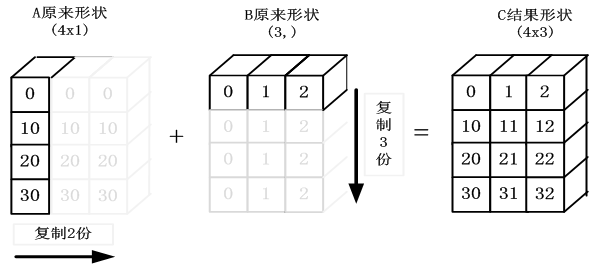## 花式索引和索引技巧

NumPy提供比常规Python序列更多的索引功能。除了通过整数和切片进行索引之外，正如我们之前看到的，数组可以由整数数组和布尔数组索引。

### 使用索引数组进行索引

>>> a = np.arange(12)**2                       # the first 12 square numbers
>>> i = np.array( [ 1,1,3,8,5 ] )              # an array of indices
>>> a[i]                                       # the elements of a at the positions i
array([ 1,  1,  9, 64, 25])
>>>
>>> j = np.array( [ [ 3, 4], [ 9, 7 ] ] )      # a bidimensional array of indices
>>> a[j]                                       # the same shape as j
array([[ 9, 16],
[81, 49]])

>>> palette = np.array( [ [0,0,0],                # black
...                       [255,0,0],              # red
...                       [0,255,0],              # green
...                       [0,0,255],              # blue
...                       [255,255,255] ] )       # white
>>> image = np.array( [ [ 0, 1, 2, 0 ],           # each value corresponds to a color in the palette
...                     [ 0, 3, 4, 0 ]  ] )
>>> palette[image]                            # the (2,4,3) color image
array([[[  0,   0,   0],
[255,   0,   0],
[  0, 255,   0],
[  0,   0,   0]],
[[  0,   0,   0],
[  0,   0, 255],
[255, 255, 255],
[  0,   0,   0]]])

### 使用布尔数组进行索引

>>> a = np.arange(12).reshape(3,4)
>>> b = a > 4
>>> b                                          # b is a boolean with a's shape
array([[False, False, False, False],
[False,  True,  True,  True],
[ True,  True,  True,  True]])
>>> a[b]                                       # 1d array with the selected elements
array([ 5,  6,  7,  8,  9, 10, 11])

>>> a[b] = 0                                   # All elements of 'a' higher than 4 become 0
>>> a
array([[0, 1, 2, 3],
[4, 0, 0, 0],
[0, 0, 0, 0]])

>>> a = np.arange(12).reshape(3,4)
>>> b1 = np.array([False,True,True])             # first dim selection
>>> b2 = np.array([True,False,True,False])       # second dim selection
>>>
>>> a[b1,:]                                   # selecting rows
array([[ 4,  5,  6,  7],
[ 8,  9, 10, 11]])
>>>
>>> a[b1]                                     # same thing
array([[ 4,  5,  6,  7],
[ 8,  9, 10, 11]])
>>>
>>> a[:,b2]                                   # selecting columns
array([[ 0,  2],
[ 4,  6],
[ 8, 10]])
>>>
>>> a[b1,b2]                                  # a weird thing to do
array([ 4, 10])

### ix_()函数

ix_函数可用于组合不同的向量，以便获得每个n-uplet的结果。例如，如果要计算从每个向量a，b和c中取得的所有三元组的所有a + b * c：

>>> a = np.arange(10).reshape(2, 5)

>>> a
array([[0, 1, 2, 3, 4],
[5, 6, 7, 8, 9]])

>>> ixgrid = np.ix_([0, 1], [2, 4])

>>> ixgrid
(array([,
]), array([[2, 4]]))

>>> ixgrid.shape, ixgrid.shape
((2, 1), (1, 2))

>>> a[ixgrid]
array([[2, 4],
[7, 9]])

>>> ixgrid = np.ix_([True, True], [2, 4])

>>> a[ixgrid]
array([[2, 4],
[7, 9]])

>>> ixgrid = np.ix_([True, True], [False, False, True, False, True])

>>> a[ixgrid]
array([[2, 4],
[7, 9]])

>>> def ufunc_reduce(ufct, *vectors):
...    vs = np.ix_(*vectors)
...    r = ufct.identity
...    for v in vs:
...        r = ufct(r,v)
...    return r

>>> ufunc_reduce(np.add,a,b,c)
array([[[15, 14, 16, 18, 13],
[12, 11, 13, 15, 10],
[11, 10, 12, 14,  9]],
[[16, 15, 17, 19, 14],
[13, 12, 14, 16, 11],
[12, 11, 13, 15, 10]],
[[17, 16, 18, 20, 15],
[14, 13, 15, 17, 12],
[13, 12, 14, 16, 11]],
[[18, 17, 19, 21, 16],
[15, 14, 16, 18, 13],
[14, 13, 15, 17, 12]]])

1

### 评论 (1)

1.